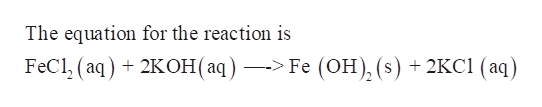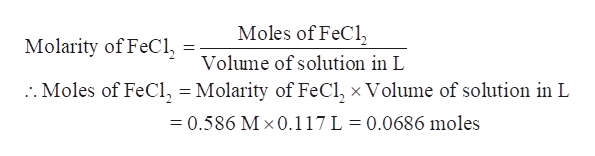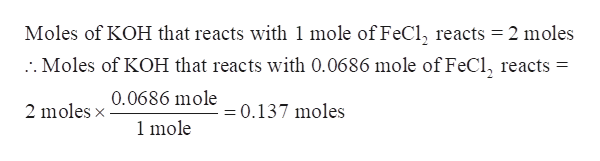# Calculate the number of milliliters of 0.800 M KOH required to precipitate all of the Fe +2 ions in 117 mL of 0.586 M FeCl2 solution as Fe(OH)2 . The equation for the reaction isFeCl2(aq) + 2KOH(aq) —-> Fe (OH)2(s) + 2KCl (aq)

Question
65 views

Calculate the number of milliliters of 0.800 M KOH required to precipitate all of the Fe +2 ions in 117 mL of 0.586 M FeCl2 solution as Fe(OH)2 . The equation for the reaction is

FeCl2(aq) + 2KOH(aq) —-> Fe (OH)2(s) + 2KCl (aq)

check_circle

Step 1

The number of millilitres of 0.800 M KOH required to precipitate all of the Fe +2 ions in 117 mL of 0.586 M FeCl2 solution as Fe(OH)2 is to be calculated.help_outlineImage TranscriptioncloseThe equation for the reaction is FeC1,(aq) + 2KОН (аq) —> Fe (ОН), (s) + 2KС1 (аq) fullscreen
Step 2

Calculate the moles of given FeCl2-

Molarity of FeCl2 = 0.586 M

Volume of FeCl2 = 117 mL = 0.117 Lhelp_outlineImage TranscriptioncloseMoles of FeCl Molarity of FeCl Volume of solution in L 2. Moles of FeCl, = Molarity of FeCl, x Volume of solution in L =0.586 M x0.117 L = 0.0686 moles fullscreen
Step 3

Step 3-

Determine the moles of KOH required reacting completely with 0.0686 moles-

As per t...help_outlineImage TranscriptioncloseMoles of KOH that reacts with 1 mole of FeCl, reacts = 2 moles .Moles of KOH that reacts with 0.0686 mole of FeCl, reacts 0.0686 mole 2 moles x =0.137 moles 1 mole fullscreen

### Want to see the full answer?

See Solution

#### Want to see this answer and more?

Solutions are written by subject experts who are available 24/7. Questions are typically answered within 1 hour.*

See Solution
*Response times may vary by subject and question.
Tagged in

### Chemistry